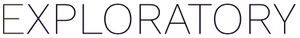# How is the importance of variables in Time Series Forecast (Prophet) Analytics View calculated?

#1

It is calculated simply as standard deviation of effect of each variable (or seasonality), which is shown in the Effect tab.
The idea is that if the model considers a variable (regressor) important, the effect of it to the final forecasted output will be larger, and we can size that effect by calculating its standard deviation.
For variables (regressors), standard deviation of effect is same as “beta” coefficient for the variable in the Prophet model.